# 一、 mskmod函数初探

mskmod - Minimum shift keying modulation
This MATLAB function outputs the complex envelope y of the modulation of the
message signal x using differentially encoded minimum shift keying (MSK)
modulation.

``````y = mskmod(x,nsamp)
y = mskmod(x,nsamp,dataenc)
y = mskmod(x,nsamp,dataenc,ini_phase)
[y,phaseout] = mskmod(...)
``````

## 1. 基础用法

``````R  = 1e1;   % The bit speed
Fc = 1e1;   % The carrier frequency
Fs = 1e2;   % the sample frequency
Ts = 1/Fs;
Upsample = Fs/R;
``````

## 2.编码

mskmod函数第三个参数是是否做数据差分编码，默认情况下，mskmod是’diff’方式进行调制，也就是默认情况下，mskmod函数认为你的输入是已经经过差分编码的

’nondiff’ 表示输入的数据是未经差分编码
’diff’ 表示输入的数据是经过差分编码的

dataenc can be either ‘diff’ for differentially encoded MSK or ‘nondiff’ for nondifferentially encoded MSK.

``````% msk差分编码
b0=1;
for i=1:N
encode_output(i)=b0*bitstream(i);%对应bk
b0=bitstream(i);
end
``````

#### msk需要的差分编码是如下图这种方式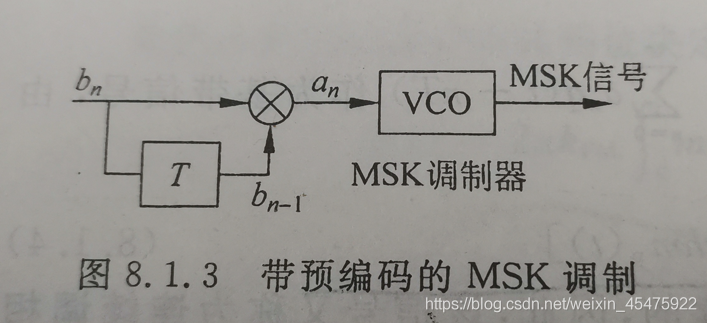``````b0=1;
for i=1:N
encode_output(i)=-b0*bitstream(i);
b0=encode_output(i);
end  %差分编码
``````

# 二、 正交调制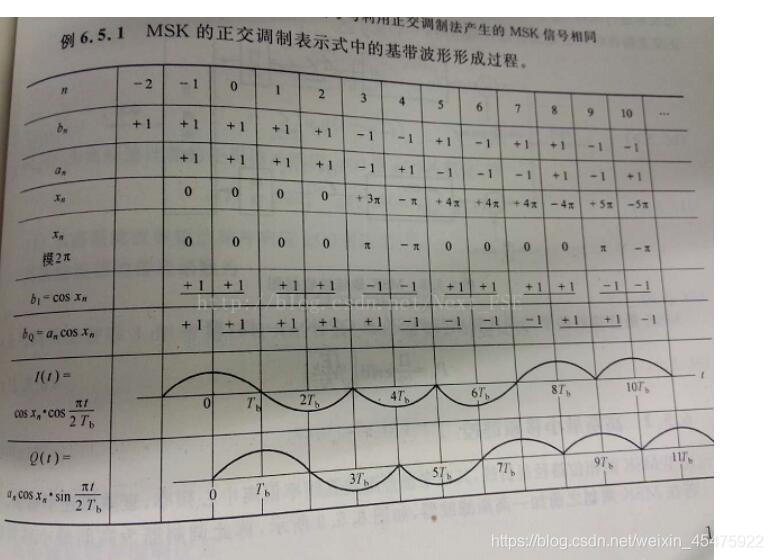mskmod函数代码为：

``````tx_data = mskmod(encode_output1,Upsample,'diff',0*pi);
``````

``````    I=[];Q=[];%奇数进I路、偶数进Q路
bitstream3 = [bitstream1(1),bitstream1];
I = bitstream3(1:2:length(bitstream3));
Q = bitstream3(2:2:length(bitstream3));

%调制
I_data=[];Q_data=[];
base_wave=-1/R:1/Fs:1/R-1/Fs;

% I_data = kron(I,cos(pi*base_wave/(2*T)));
n = -Upsample:length(I)*2*Upsample-Upsample-1;
cos_I = cos(pi*n*Ts*R./2);
I_data_temp = kron(I,ones(1,2*Upsample)).* cos_I;
I_data = I_data_temp(Upsample+1:end+Upsample*(mod(N,2)-1));%取出零时刻的值
%此处考虑了总共有奇数个bit需要传输的情况，实际上不需要考虑

% Q_data = kron(Q,sin(pi*base_wave/(2*R)));
n = 0:length(Q)*2*Upsample-1;
sin_Q = sin(pi*n*Ts*R./2);
Q_data_temp = kron(Q,ones(1,2*Upsample)) .* sin_Q;
Q_data = Q_data_temp(1:end-Upsample*mod(N,2));%%取出零时刻的值
%此处考虑了总共有奇数个bit需要传输的情况，实际上不需要考虑

% Q路延迟半个符号
number_delay=length(base_wave)/2;
% Q_data1=[zeros(1,number_delay),Q_data(1:length(Q_data)-number_delay)];

% 载波信息
bit_t=0:1/Fs:N/R-1/Fs;
I_carrier=cos(2*pi*Fc*bit_t);
Q_carrier=sin(2*pi*Fc*bit_t);

% 调制
I_band = I_data.*I_carrier;
Q_band = Q_data.*Q_carrier;

% 两路合成一路
MSK_signal = I_band + 1i*Q_band;
``````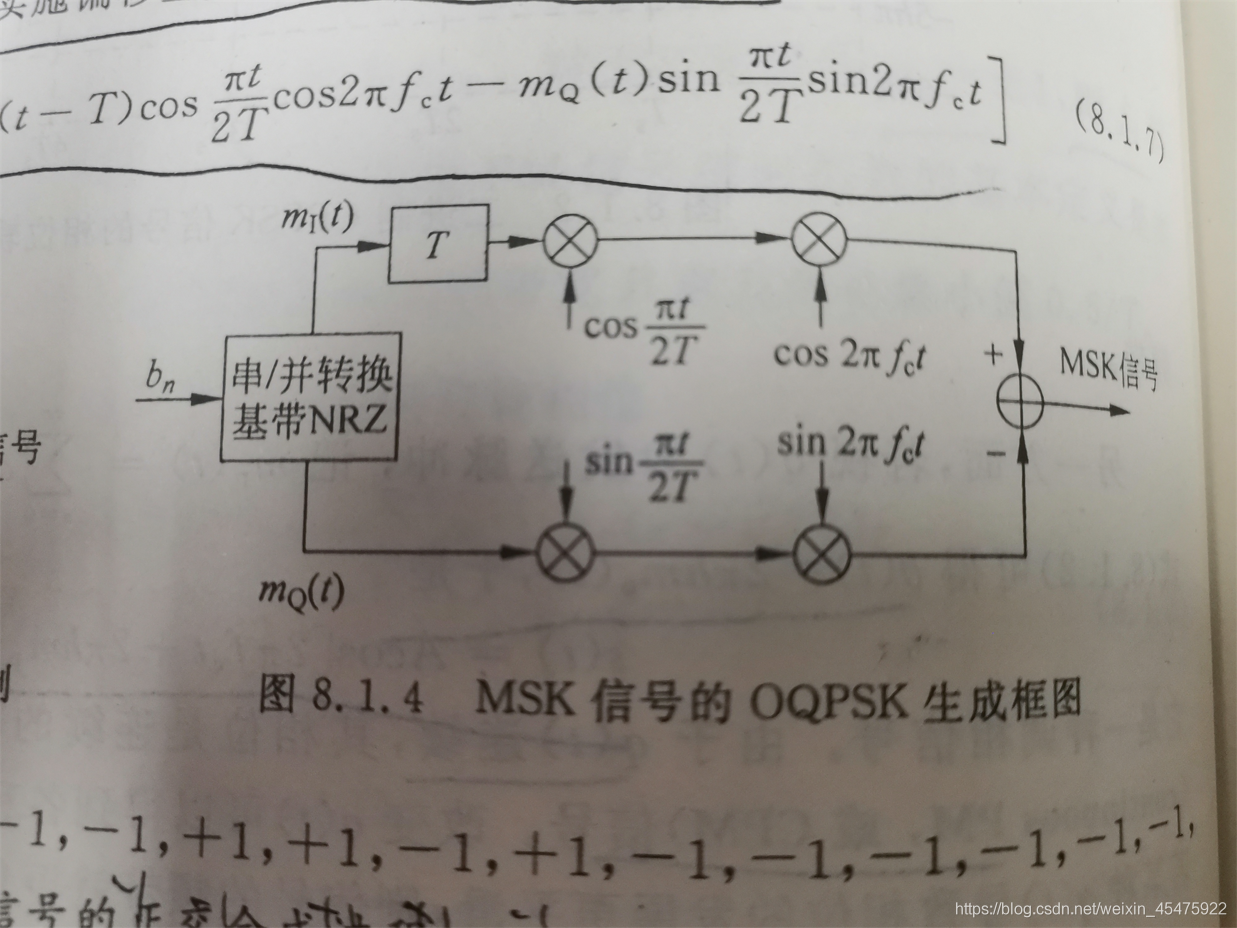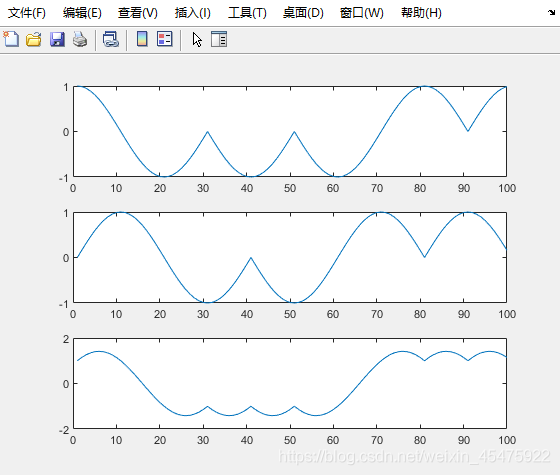# 3. 解调

``````    I_output = MSK_receive.*I_carrier;
I_filter_ouput_delay=[I_output(number_delay+1:end) zeros(1,number_delay)];
``````

``````%第二次本地相关解调
I_dected = real(I_filter_ouput_delay).*cos_I(2*number_delay+1:end);
Q_dected = imag(Q_output).*sin_Q;

%积分判决
for i=1:round(N/2)
I_recover(i) = sign(sum(I_dected((i-1)*2*number_delay+1:i*2*number_delay)));
Q_recover(i) = sign(sum(Q_dected((i-1)*2*number_delay+1:i*2*number_delay)));
end

% 合并IQ两路
bit_recover = zeros(1,N);
bit_recover(1:2:end) = Q_recover;
bit_recover(2:2:end) = I_recover;
bit_recover = (bit_recover+1) / 2;
``````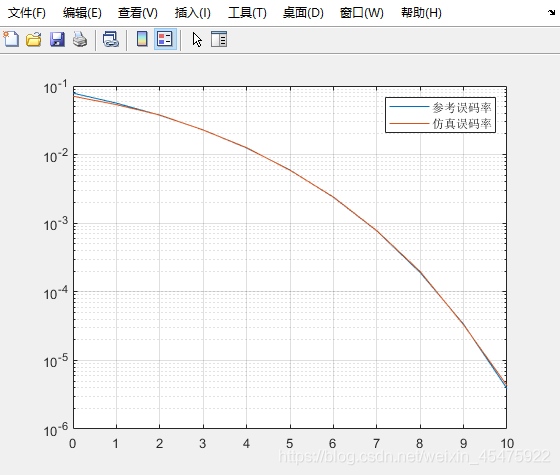# 完整的代码我放在这里：

``````%%%%%%%%%%%%%%%%%%%%%  MSK调制解调 %%%%%%%%%
%%%%% data:2021年8月10日  author:卡布基诺加勺糖 %%%%%%

%%%%%程序说明
%MSK调制解调器的仿真
%调制方式：MSK 编码：差分编码（正交调制等效于差分编码？？）
%噪声类型：线性高斯白噪声
%解调解调方式：采用正交调制、相干解调

%%%%%仿真环境
% 软件版本：MATLAB2019a

%% %%%%%%%%%%%%%%%%% 参数设定 %%%%%%%%%%%%%%%%%%%%%%
clc;clear all;
tic
R  = 1e1;   % The bit speed
Fc = 1e1; % The carrier frequency
Fs = 1e2;   % the sample frequency
Ts = 1/Fs;
Upsample = Fs/R;

%% %%%%%%%%%%%%%%%%% 主程序 %%%%%%%%%%%%%%%%%%%%%%
EbN0_loop   = [0   2   4   6   8   ]   ;   % signal to noise rate
N_loop      = [1e4 5e4 1e5 5e5 1e6 ];          %循环点数
for loop=1:length(EbN0_loop)
%%%%%%%%%%%%%%%%%%% 信源 %%%%%%%%%%%%%%%%%%%%%%
%     loop=1; % use for debug
% 生成信息序列
N = N_loop(loop);
bitstream=randi([0,1],1,N);  % randomly generate the bitstream
bitstream = [1 1 1 0 0 1 0 1 1 0];
bitstream1=2*bitstream-1;  % 双极性

%%%%%%%%%%%%%%%%%%% 编码 %%%%%%%%%%%%%%%%%%%%%%
% b0=1;
% for i=1:N
%     encode_output(i)=-b0*bitstream1(i);
%     b0=encode_output(i);
% end  %dpsk差分编码

b0=1;
for i=1:N
encode_output(i)=b0*bitstream1(i);%对应bk
b0=bitstream1(i);
end  % msk差分编码

%%%%%%%%%%%%%%%%%%% 调制 %%%%%%%%%%%%%%%%%%%%%%
% % 用matlab已有函数mskmod进行调制
%     encode_output1 = (encode_output + 1)/2;
%     tx_data = mskmod(encode_output1,Upsample,'diff',0*pi);
% % mskmod: the elements of x must be 0 or 1

% 自己写的正交调制
I=[];Q=[];%奇数进I路、偶数进Q路
bitstream3 = [bitstream1(1),bitstream1];
I = bitstream3(1:2:length(bitstream3));
Q = bitstream3(2:2:length(bitstream3));

%调制
I_data=[];Q_data=[];
base_wave=-1/R:1/Fs:1/R-1/Fs;

n = -Upsample:length(I)*2*Upsample-Upsample-1;
cos_I = cos(pi*n*Ts*R./2);
I_data_temp = kron(I,ones(1,2*Upsample)).* cos_I;
I_data = I_data_temp(Upsample+1:end+Upsample*(mod(N,2)-1));%取出零时刻的值
%此处考虑了总共有奇数个bit需要传输的情况，实际上不需要考虑

n = 0:length(Q)*2*Upsample-1;
sin_Q = sin(pi*n*Ts*R./2);
Q_data_temp = kron(Q,ones(1,2*Upsample)) .* sin_Q;
Q_data = Q_data_temp(1:end-Upsample*mod(N,2));%%取出零时刻的值
%此处考虑了总共有奇数个bit需要传输的情况，实际上不需要考虑

% Q路延迟半个符号
number_delay=length(base_wave)/2;

% 载波信息
bit_t=0:1/Fs:N/R-1/Fs;
I_carrier=cos(2*pi*Fc*bit_t);
Q_carrier=sin(2*pi*Fc*bit_t);

% 调制
I_band = I_data.*I_carrier;
Q_band = Q_data.*Q_carrier;

% 两路合成一路
MSK_signal = I_band + 1i*Q_band;

%%%%%%%%%%%%%%%%%%%%%%% 信道 %%%%%%%%%%%%%%%%%%%%%%%
% 加入噪声
EbN0 = EbN0_loop(loop);
snr  = EbN0 - 10*log10(Upsample);

%%%%%%%%%%%%%%%%%%% 解调 %%%%%%%%%%%%%%%%%%%%%%
I_filter_ouput_delay=[I_output(number_delay+1:end) zeros(1,number_delay)];
I_dected = real(I_filter_ouput_delay).*cos_I(2*number_delay+1:end);

Q_dected = imag(Q_output).*sin_Q;

for i=1:round(N/2)
I_recover(i) = sign(sum(I_dected((i-1)*2*number_delay+1:i*2*number_delay)));
Q_recover(i) = sign(sum(Q_dected((i-1)*2*number_delay+1:i*2*number_delay)));
end

bit_recover = zeros(1,N);
bit_recover(1:2:end) = Q_recover;
bit_recover(2:2:end) = I_recover;
bit_recover = (bit_recover+1) / 2;

error_rate(loop) = sum(abs(bit_recover - bitstream)) / N ;

end

%%%%%%%%%%%%%%%%%画图%%%%%%%%%%%%%%%%%

%save('error_rate.mat','error_rate');
toc
disp('save success')

figure()
ber_standard = berawgn(EbN0_loop,'msk','on','coherent');
semilogy(EbN0_loop,ber_standard);
hold on
semilogy(EbN0_loop,error_rate);
legend('参考误码率','仿真误码率')
grid on
``````

# 写在后面

.
.

1. 先说第二次自相关和第二个理论上的低通滤波：
这里其实我是用累加来代替低通滤波了，高频项在累加过程中，sin或cos函数转了一圈，积分为0，因此变相的完成了低通滤波
2. 第一次与本地载波自相关后我没有加入低通滤波
其实这里我本来在代码中写了低通滤波器的，并且仿真得到的结果与理论值也是一样的，但是我突然想，如果我去掉这个低通滤波，我只用一个低通滤波可不可以？
这里以I路的公式为例，如果第一次相关后不做低通滤波，那么高频项会一直保留，在第二次相关时产生高频。一开始我直观的想，我进行累加，第二次相关的2到5项都会积分得到零，所以仿真中我去掉了第一个低通滤波，进行仿真，结果和不进行第一次低通滤波的误码率相同。
但是实际上，只有第二项可以在累加求和中得到零，其他项由于存在Wc频率的sin或cos，导致积分不为零，因此，从理论上讲这会影响我的误码率，但是仿真结果是正确的，而且我也考虑了Wc和Ts的倍数关系，我将两者设置成不是整倍数的数值，仿真结果也是正确的，这让我很费解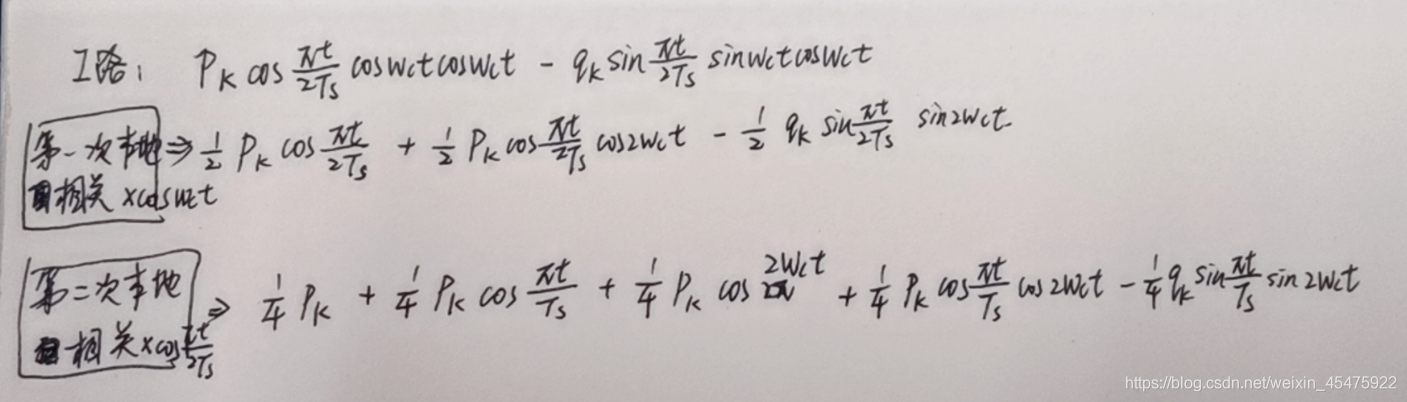# 有没有大佬解惑，求大佬解惑！！

### Spark | 读取Hive表数据写入MySQL_点滴笔记的博客-程序员宅基地

import java.sql.Connectionimport scala.collection.mutable.ArrayBufferobject JdbcTemplateUtil extends Serializable { /** * 单条操作 * @param sql * @param params */ def executeSql(conn: Connection, sql: String, params: ...

### Centos8搭建Mosquitto（三）workerman/mqtt+workerman/mysql完成MQTT订阅消息持久存储Mysql数据库_老刘307的博客-程序员宅基地

Workerman是什么？Workerman是一款纯PHP开发的开源高性能的PHP socket 服务框架。Workerman不是重复造轮子，它不是一个MVC框架，而是一个更底层更通用的socket服务框架，你可以用它开发tcp代理、梯子代理、做游戏服务器、邮件服务器、ftp服务器、甚至开发一个php版本的redis、php版本的数据库、php版本的nginx、php版本的php-fpm等等。Workerman可以说是PHP领域的一次创新，让开发者彻底摆脱了PHP只能做WEB的束缚。实际上Worker_workerman/mqtt

### elasticsearch---search in depth之full-text search_2B铅笔水果橡皮的博客-程序员宅基地

full-text中两个关键因素：analyzed和relevence

### Linux文件共享服务之NFS_fns共享_谢公子的博客-程序员宅基地

NFS(Network File System) 网络文件系统，是FreeBSD支持的文件系统中的一种，它允许网络中的计算机之间通过TCP/IP网络共享资源。在NFS的应用中，本地NFS的客户端应用可以透明地读写位于远端NFS服务器上的文件，就像访问本地文件一样。NFS主要用于LInux与Linux之间进行文件系统共享。简单的来说：它就是是可以透过网络，让不同的主机、不同的操作系统可以共享存储..._fns共享

### ROS入门之Cmakelist说明_catkin_include_dirs_Bourne_Boom的博客-程序员宅基地

Cmakelisthttp://wiki.ros.org/catkin/CMakeLists.txt1 Overall Structure and OrderingYour CMakeLists.txt file MUST follow this format otherwise your packages will not build correctly. The order in ..._catkin_include_dirs